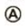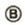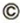Chapter 5, Problem 77AP

Chapter
Section
Textbook Problem

A child’s pogo slick (Fig. P5.77) stores energy in a spring (k = 2.50 × 104 N/m). At position(x1 = −0.100 m), the spring compression is a maximum and the child is momentarily at rest. At position(x = 0), the spring is relaxed and the child is moving upward. At position, the child is again momentarily at rest at the top of the jump. Assuming that the combined mass of child and pogo stick is 25.0 kg, (a) calculate the total energy of the system if both potential energies are zero at x = 0, (b) determine x2, (c) calculate the speed of the child at x = 0, (d) determine the value of x for which the kinetic energy of the system is a maximum, and (e) obtain the child’s maximum upward speed.Figure P5.77

(a)

To determine
The total energy of the system if both potential energies are zero at x=0 .

Explanation

Given Info:

The combined mass of child and pogo stick is 25.0kg .

The maximum spring compression is 0.100m .

The spring constant is 2.50×104N/m .

Formula to calculate the total energy of the system is,

E=(KE+PEg+PEs)A

• PEg is the potential energy due to gravity
• PEs is the spring potential energy
• KE is the kinetic energy

Since the child is in rest at the point A, the kinetic energy of the child is zero at A.

Thus,

E=(PEg+PEs)A=mgx1+12kx12

• x1 is the compression of the spring
• m is the combined mass of child and the pogo stick
• g is acceleration due to gravity
• k is the spring constant

Substitute 25

(b)

To determine
x2 .

(c)

To determine
The speed of the child at x=0 .

(d)

To determine
To determine the value for x for which the kinetic energy of the system is maximum.

(e)

To determine
The maximum upward speed of the child.

Still sussing out bartleby?

Check out a sample textbook solution.

See a sample solution

The Solution to Your Study Problems

Bartleby provides explanations to thousands of textbook problems written by our experts, many with advanced degrees!

Get Started

Find more solutions based on key concepts